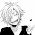## Justin's Proportion Problems

1) If 3 apples cost \$6, how much would 12 apples cost?
let x be the cost of 12 apples
Steps= ratio table, word ratio, find x
The cost of the 12 apples is     24  .

2) 3 pounds cost \$15. How many pounds can you buy for \$45?
let x be the cost of how many pounds u get for \$45.
Steps=ratio table, word ratio, find x

there are   9 pounds for \$45.

3) Darrel drove 187 in 3 hours. What was Darrel's average rate of speed in miles per hour?
let x be Darrel's average speed in miles
Steps=ratio table, word ratio, find x

Darrel's average rate of speed in miles is about 62.33

1:44 PM

#### 1 comment :

1.Justin why did you answer your question we suppose to answer yours not you. >.<